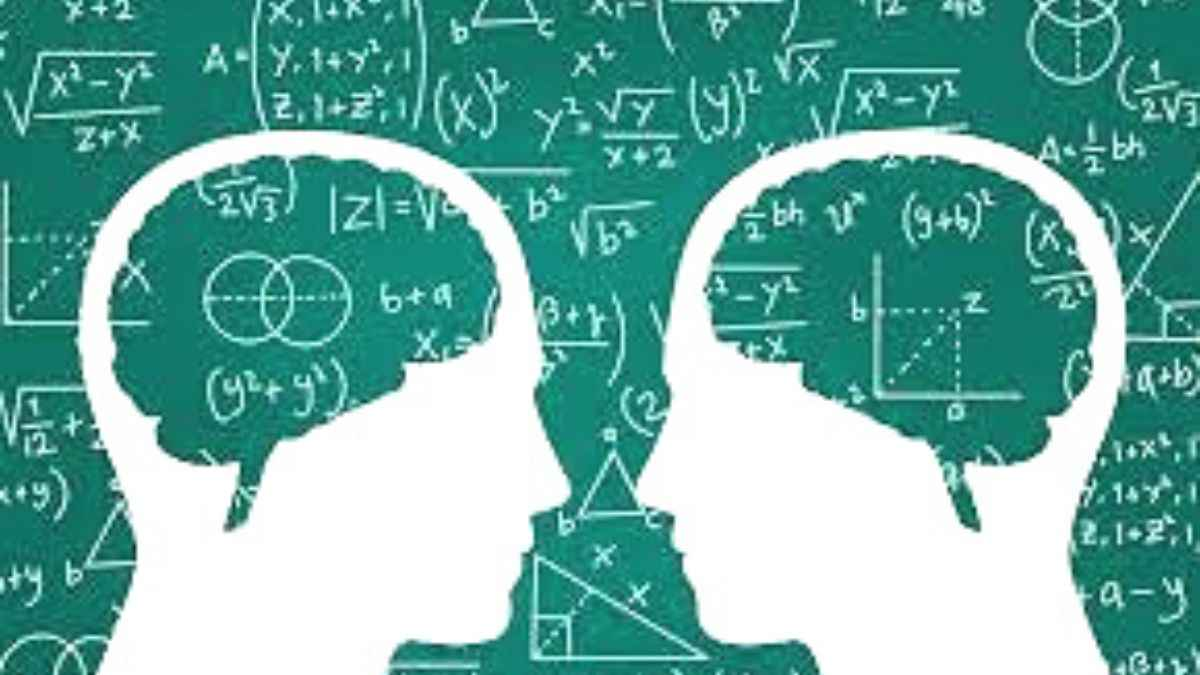# These math riddles are fun, challenging, addictive and what not! Give them a quick try.

Math can be fun too. Don't believe us? Try these math riddles!Have you ever thought how the world would be without math? How would you have calculated your favorite fruits? How would architects have measured the right dimensions? In short, how would the world run?

Well, if math is so essential for survival, why do many people hate the subject? If the world would have not run properly without math, why don’t people feel grateful for the subject?

This is because math is often considered as a tough subject. Many people have this conditioned fear of math that they have cultivated their entire childhood. Many people feel the urge to skip every math problem that comes their way or grab a calculator to solve simple mathematical problems.

Today, we are here to bust every myth and misconception, thereby making math not only simple but also enjoyable.

Try these math riddles, and you may change your perception regarding the subject.

## MATH RIDDLES:

### MATH RIDDLE 1:

Add the number to the number itself and then multiply by 4. Again divide the number by 8 and you will get the same number once more. Which is that number?

### MATH RIDDLE 2:

At the time of shipping, Peter can place 10 small boxes or 8 large boxes into a carton. A total of 96 boxes were sent in one shipment. The number of small boxes was less than large boxes. What is the total number of cartons he shipped?

### MATH RIDDLE 3:

I am a three-digit number. My second digit is 4 times bigger than the third digit. My first digit is 3 less than my second digit. Who am I?

### MATH RIDDLE 4:

Eggs are \$0.12 a dozen. How many eggs can you get for a dollar?

### MATH RIDDLE 5:

How many sides does a circle have?

Yes, we can hear that tiny voice in your gut that is asking for answers without any delays. Here you go!

MATH RIDDLE 1:

Add the number to the number itself and then multiply by 4. Again divide the number by 8 and you will get the same number once more. Which is that number?

Any number

MATH RIDDLE 2:

At the time of shipping, Peter can place 10 small boxes or 8 large boxes into a carton. A total of 96 boxes were sent in one shipment. The number of small boxes was less than large boxes. What is the total number of cartons he shipped?

11 cartons

4 small boxes (4*10 = 40 boxes)

7 large boxes (7*8 = 56 boxes)

So 96 boxes and 11 total cartons

MATH RIDDLE 3:

I am a three-digit number. My second digit is 4 times bigger than the third digit. My first digit is 3 less than my second digit. Who am I?

141

MATH RIDDLE 4:

Eggs are \$0.12 a dozen. How many eggs can you get for a dollar?

100 eggs, at one penny each

MATH RIDDLE 5:

How many sides does a circle have?

Two. Inside and Outside.

Weren’t these fun? Well, we are sure they were!

These brain teasers are so fun, they will make your day!

Get the latest General Knowledge and Current Affairs from all over India and world for all competitive exams.
खेलें हर किस्म के रोमांच से भरपूर गेम्स सिर्फ़ जागरण प्ले पर